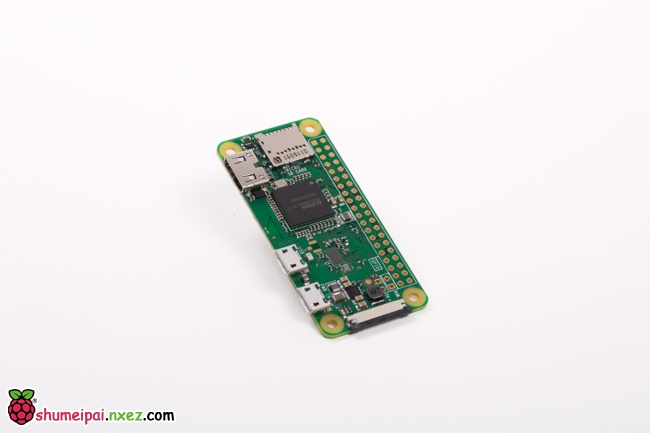# 树莓派是如何免疫 Meltdown 和 Spectre 漏洞的```t = a+b
u = c+d
v = e+f
w = v+g
x = h+i
y = j+k```

```t, u = a+b, c+d
v, w = e+f, v+g
x, y = h+i, j+k```

```t, u = a+b, c+d
v    = e+f                   # second pipe does nothing here
w, x = v+g, h+i
y    = j+k```

```t = a+b
u = c+d
v = e+f
x = h+i
w = v+g
y = j+k```

```t, u = a+b, c+d
v, x = e+f, h+i
w, y = v+g, j+k```

```t = a+b
u = t+c
v = u+d
if v:
w = e+f
x = w+g
y = x+h```

```t = a+b
u = t+c
v = u+d
w_ = e+f
x_ = w_+g
y_ = x_+h
if v:
w, x, y = w_, x_, y_```

```t, w_ = a+b, e+f
u, x_ = t+c, w_+g
v, y_ = u+d, x_+h
if v:
w, x, y = w_, x_, y_```

```a = mem
b = mem```

```a = mem    # 100ns delay, copies mem[0:15] into cache
b = mem    # mem is in the cache```

「边信道攻击是基于从密码系统的物理实现获得的信息的任何攻击，而不是算法中的蛮力或理论弱点（相较于密码分析学）。例如，定时信息、功耗、电磁泄漏甚至声音都可以提供额外的信息源，这些信息可被用来破解系统。」

Spectre 和 Meltdown 属于边信道攻击，通过定时来观察缓存中是否有另一个可访问的位置，以推断内存位置的内容，这些内容通常不应该被访问。

```t = a+b
u = t+c
v = u+d
if v:
w = kern_mem[address]   # if we get here, fault
x = w&0x100
y = user_mem[x]```

```t, w_ = a+b, kern_mem[address]
u, x_ = t+c, w_&0x100
v, y_ = u+d, user_mem[x_]

if v:
# fault
w, x, y = w_, x_, y_      # we never get here```

• v 是零，所以非法读取的结果不会被提交给 w
• v 是非零，但在读取结果被提交给 w 之前发生了错误

`v, y_ = u+d, user_mem[x_]`